Next: Expected Count Rates Up: FEASIBILITY: XMA+PSPC Previous: FEASIBILITY: XMA+PSPC

# Minimum detectable count rates

The minimum detectable count rate for the PSPC depends on the background count rate and the effective size of an unresolved source (i.e., it depends on the off-axis angle). The minimum detectable count rate,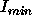, can be estimated from the formula: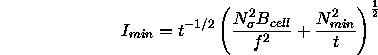where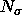is the required significance threshold (i.e., signal-to-noise),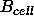is the background count rate in the ``detection cell'', f is the fraction of the total source count included in the ``detection cell'',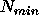is the minimum number of photons required to constitute a detection and t is the integration time.

Note that the effective point response function of the PSPC depends on energy (cf., § 4.2.2) and hence on the spectrum of the considered source as well as the position of the source in the field of view. For the sensitivity calculations a circular detection cell of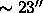radius, an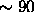percent fraction of all source photons in the detect cell, a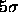confidence level of detection as well as a minimum of number of 10 counts per source have been assumed. Because the PSPC background depends sensitively on energy (cf., § 7.8.2), two sets of calculations are given, one applicable to the total band (0.1 - 2.4 keV), the other to the hard band (0.4 - 2.4 keV) only, where the overall background is significantly reduced. In Figure 10.1, the minimum detectable PSPC count rate is plotted as a function of exposure time for a low, medium and high level of mean detector background in the total band and hard band respectively. As is obvious from Figure 10.1the PSPC becomes background-limited for exposure times around 10 ks depending on the background level (and the chosen detection cell size). However, the transition between the photon- and background limited regime is rather smooth; note that Figure 10.1only applies to on-axis observations, for off-axis observations larger detect cell sizes and consequently larger background values must be used.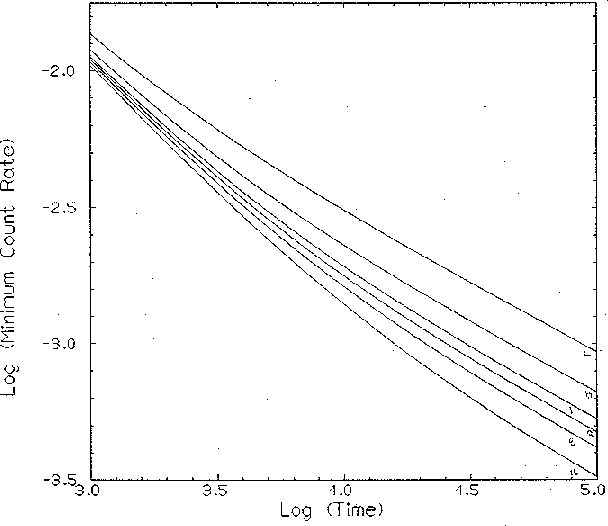Next: Expected Count Rates Up: FEASIBILITY: XMA+PSPC Previous: FEASIBILITY: XMA+PSPC

Michael Arida
Tue Jun 11 16:18:41 EDT 1996